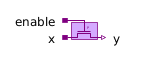C_Capacitor - MapleSim Help

C_Capacitor

Ideal linear electrical capacitorDescription The C_Capacitor component models a linear capacitor. The Capacitance C is allowed to be positive, zero, or negative.Equations $i={i}_{p}=-{i}_{n}=C\frac{\mathrm{dv}}{\mathrm{dt}}$ $v={v}_{p}-{v}_{n}$Variables

 Name Units Description Modelica ID $v$ $V$ Voltage drop between the two pins $\left({v}_{p}-{v}_{n}\right)$ v $i$ $A$ Current flowing from pin p to pin n iConnections

 Name Description Modelica ID $p$ Positive pin p $n$ Negative pin nParameters

 Name Default Units Description Modelica ID $C$ $0$ $F$ Capacitance C $\mathrm{IC}$ $0$ $V$ Initial value IC $\mathrm{UIC}$ $\mathrm{false}$ Use initial conditions: true, if initial condition is used UICModelica Standard Library The component described in this topic is from the Modelica Standard Library. To view the original documentation, which includes author and copyright information, click here.